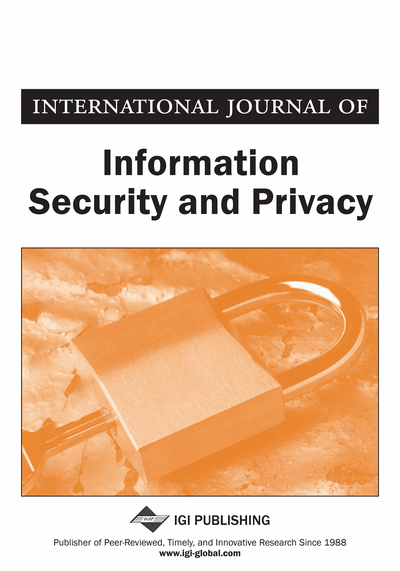# Image Encryption Algorithm Based on a Novel 4D Chaotic System

DOI: 10.4018/IJISP.2021100107
OnDemand:
(Individual Articles)
Available
\$29.50
No Current Special Offers

## Abstract

In this paper, a novel four-dimensional chaotic system has been created, which has characteristics such as high sensitivity to the initial conditions and parameters. It also has two a positive Lyapunov exponents. This means the system is hyper chaotic. In addition, a new algorithm was suggested based on which they constructed an image cryptosystem. In the permutation stage, the pixel positions are scrambled via a chaotic sequence sorting. In the substitution stage, pixel values are mixed with a pseudorandom sequence generated from the 4D chaotic system using XOR operation. A simulation has been conducted to evaluate the algorithm, using the standardized tests such as information entropy, histogram, number of pixel change rate, unified average change intensity, and key space. Experimental results and performance analyses demonstrate that the proposed encryption algorithm achieves high security and efficiency.
Article Preview
Top

## 2. Literature Review

In recent years many researchers used chaotic systems to image encryption. Some related work is explained below:

Tianetal(2010) presented a new autonomous four-dimension hyper chaotic system, where the basic dynamical behaviours are investigated. The system possess one equilibrium point and four Lyapunov exponents obtain as LE1= 1.6170, LE2 =0.1123, LE3= 0, LE4= -12.8245,where the Lyapunov dimension has fractional nature calculated as 3.1348.

Baojie Zhang and Hongxing Li (2013) proposed an autonomous non-linear four-dimension hyper chaotic system on basis of Lorenz system. The dynamical behaviour of system was investigated with equilibrium Lyapunov exponents and dissipation which demonstrate that the new system possesses rich dynamical behaviour. Lyapunov exponents obtains as LE1= 1.156884, LE2=0.720394, LE3= 0.026702 and LE4 = -44.999849.

## Complete Article List

Search this Journal:
Reset
Volume 17: 1 Issue (2023)
Volume 16: 4 Issues (2022): 2 Released, 2 Forthcoming
Volume 15: 4 Issues (2021)
Volume 14: 4 Issues (2020)
Volume 13: 4 Issues (2019)
Volume 12: 4 Issues (2018)
Volume 11: 4 Issues (2017)
Volume 10: 4 Issues (2016)
Volume 9: 4 Issues (2015)
Volume 8: 4 Issues (2014)
Volume 7: 4 Issues (2013)
Volume 6: 4 Issues (2012)
Volume 5: 4 Issues (2011)
Volume 4: 4 Issues (2010)
Volume 3: 4 Issues (2009)
Volume 2: 4 Issues (2008)
Volume 1: 4 Issues (2007)
View Complete Journal Contents Listing